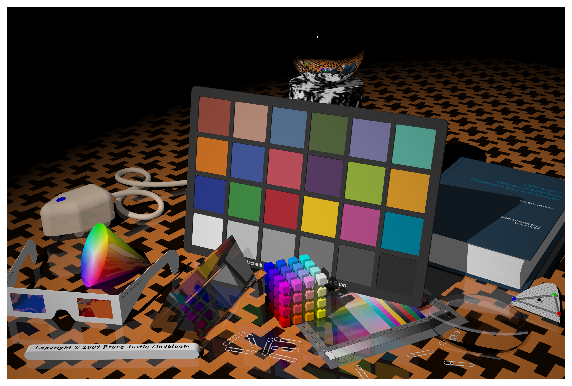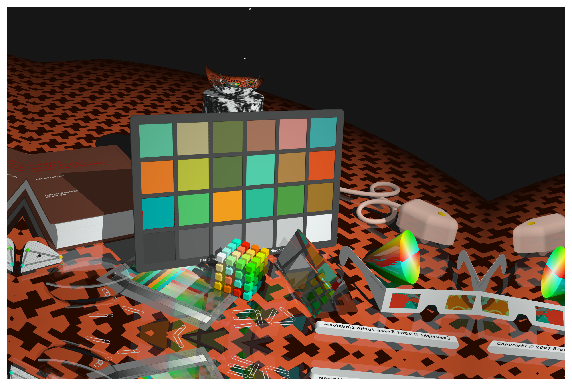# Working with non-8-bit images¶

This example shows how you can augment 16-bit TIFF images. 16-bit images are used in satellite imagery. The following technique could also be applied to all non-8-bit images (i.e., 24-bit images, 32-bit images. etc.).

### Import the required libraries¶

import random

import cv2
from matplotlib import pyplot as plt

import albumentations as A


### Define the visualization function¶

def visualize(image):
# Divide all values by 65535 so we can display the image using matplotlib
image = image / 65535
plt.figure(figsize=(10, 10))
plt.axis('off')
plt.imshow(image)


### Read the 16-bit TIFF image from the disk¶

# The image is taken from http://www.brucelindbloom.com/index.html?ReferenceImages.html
image = cv2.cvtColor(image, cv2.COLOR_BGR2RGB)
visualize(image)Note: OpenCV may read incorrectly some TIFF files. Consider using tifffile - https://github.com/blink1073/tifffile

### Define an augmentation pipeline that works with 16-bit TIFF images¶

Under the hood, Albumentations supports two data types that describe the intensity of pixels: - np.uint8, an unsigned 8-bit integer that can define values between 0 and 255. - np.float32, a floating-point number with single precision. For np.float32 input, Albumentations expects that value will lie in the range between 0.0 and 1.0.

Albumentations has a dedicated transformation called ToFloat that takes a NumPy array with data types such as np.uint16, np.uint32, etc. (so any datatype that used values higher than 255 to represent pixel intensity) and converts it to a NumPy array with the np.float32 datatype. Additionally, this transformation divides all input values to lie in the range [0.0, 1.0]. By default, if the input data type is np.uint16, all values are divided by 65535, and if the input data type is np.uint32, all values are divided by 4294967295. You can specify your divider in the max_value parameter.

The augmentation pipeline for non-8-bit images consists of the following stages:

• First, you use the ToFloat transform to convert an input image to float32. All values in the converted image will lie in the range [0.0, 1.0].
• Then you use all the necessary image transforms.
• Optionally you could use the FromFloat transform at the end of the augmentation pipeline to convert the image back to its original data type.
transform = A.Compose([
A.ToFloat(max_value=65535.0),

A.RandomRotate90(),
A.Flip(),
A.OneOf([
A.MotionBlur(p=0.2),
A.MedianBlur(blur_limit=3, p=0.1),
A.Blur(blur_limit=3, p=0.1),
], p=0.2),
A.ShiftScaleRotate(shift_limit=0.0625, scale_limit=0.2, rotate_limit=45, p=0.2),
A.OneOf([
A.OpticalDistortion(p=0.3),
A.GridDistortion(p=0.1),
], p=0.2),
A.HueSaturationValue(hue_shift_limit=20, sat_shift_limit=0.1, val_shift_limit=0.1, p=0.3),

A.FromFloat(max_value=65535.0),
])


We fix the random seed for visualization purposes, so the augmentation will always produce the same result. In a real computer vision pipeline, you shouldn't fix the random seed before applying a transform to the image because, in that case, the pipeline will always output the same image. The purpose of image augmentation is to use different transformations each time.

random.seed(7)
augmented = transform(image=image)
visualize(augmented['image'])